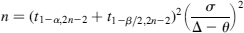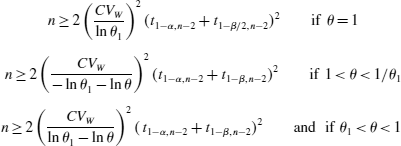## Don’t use the formula by Chow, Shao, Wang! [Power / Sample Size]

Hi David & Darya,

❝ For some reason, I can't see the image with the formula that you put on the first post.

I uploaded a copy. Should be visible by now.

❝ ❝ The only thing about what I'm not sure is CV. Because in formula that I used this is intra-subject variability, but in PowerTOST() this is coefficient of variation as ratio. In calculations in both cases I used СV - 0.3.

❝ Within subject standard deviation and within subject CV are different parameters. Nevertheless, I think that this is not the only reason for such a big difference.

Chow used the standard deviation, whilst in Power.TOST the CV (fraction, not in %!) is used. However, no big difference since $$CV_w = \sqrt{e^{s_{w}^{2}} - 1}$$ and the other way ’round $$s_w = \sqrt{\log{(CV_{w}^{2}) + 1}}$$. In Power.TOST for convenience you can use se2CV(foo) for the former and CV2se(foo) for the latter.

❝ There is a sentence in one of the articles that is quoted on SampleNTOST formula that may clarify this issue: […]

❝ So by reading this, I am not sure if Chow formula might be appropriate to calculate sample size for BABE trials.I think it’s crap. The formula Darya posted (10.2.6) of p.259 of the book gives the impression that n is the total sample size. The text continues with:

Since the above equations do not have an explicit solution, for convenience, for a 2 × 2 crossover design, the total sample size needed to achieve a power of 80% or 90% at 5% level of significance with various combinations of ε and δ is given in Table 10.2.1.

However, in the example which follows on p.260 we read:

By referring to Table 10.2.1, a total of 24 subjects per sequence is needed in order to achieve an 80% power at the 5% level of significance.

(my emphases)

❝ […] Perhaps dlabes might clarify this, since he is the master that we all should thank for the amazing PowerTOST packageDetlew is on vacation. Some clarifications:

Zhang1
(5) where n = sample size per sequence(9) with a correction term cHauschke et al.2Chow & Liu (5.4.10)3Using the formulas of Zhang or Chow & Liu, you get the sample size / sequence. To obtain the total, multiply by 2, which is already done it the right-hand side of Hauschke’s formulas.

Comparison with the references:
Table 2, untransformed data, 90% Power, Δ 0.2, σ 0.3, ∕θ 0.05 (p.5371): 37 / sequence

library(PowerTOST) sampleN.TOST(CV=se2CV(0.3), theta0=0.05, theta1=-0.2, theta2=0.2,              logscale=FALSE, targetpower=0.90,              print=FALSE)[["Sample size"]]/2  37Table 5.4.1, untransformed data, 80% Power, Δ 0.2μR, α 0.05 (p.1583): 52

library(PowerTOST) sampleN.TOST(CV=0.30, theta0=0.05, theta1=-0.2, theta2=0.2,              logscale=FALSE, targetpower=0.80,              print=FALSE)[["Sample size"]]  52Table 5.1, log-transformed data, 80% Power, (θ1, 1∕θ1) = (0.80, 1.25), θ 0.95, α 0.05 (p.1132): 40

library(PowerTOST) sampleN.TOST(CV=0.30, theta0=0.95, theta1=0.8, theta2=1.25,              targetpower=0.80,              print=FALSE)[["Sample size"]]  40Stop using the formula given by Chow, Shao, Wang! Sample sizes are way too small – which compromises power. If you used it in the past for 80% power – if all assumptions (CV, θ0) turned out to be “correct” – substantially more than 20% of studies should have failed. If not, you were lucky!

1. Zhang P. A Simple Formula for Sample Size Calculation in Equivalence Studies. J Biopharm Stat. 2003;13(3):529-38. doi:10.1081/BIP-120022772
2. Hauschke D, Steinijans V, Pigeot I. Bioequivalence Studies in Drug Development. Chichester: John Wiley; 2007. p.116. doi:10.1002/9780470094778
3. Chow S-C Liu J-p. Design and Analysis of Bioavailability and Bioequivalence Studies.
Boca Raton: Chapman & Hall/CRC Press; 3rd ed. 2009. p.157. doi:10.1201/9781420011678

Dif-tor heh smusma 🖖🏼 Довге життя Україна!Helmut SchützThe quality of responses received is directly proportional to the quality of the question asked. 🚮
Science QuotesIng. Helmut Schütz